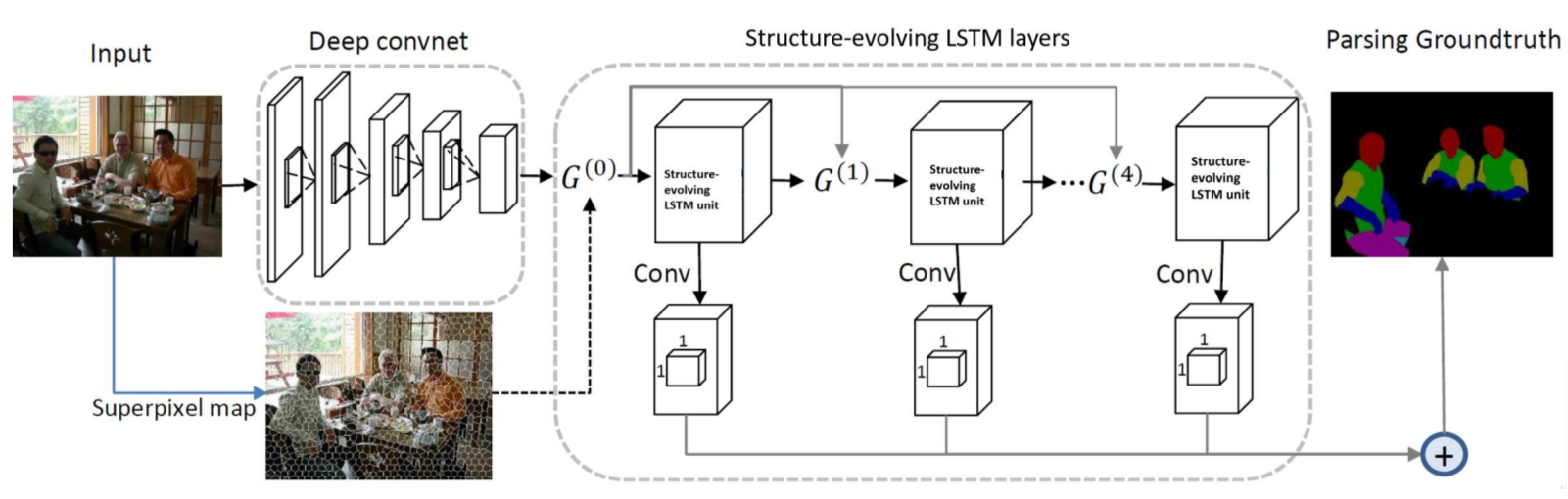CVPR 2017
Interpretable Structure-Evolving LSTM
Xiaodan Liang, Liang Lin*, Xiaohui Shen, Jiashi Feng, Shuicheng Yan, and Eric Xing
CVPR 2017

Abstract

This paper develops a general framework for learning interpretable data representation via Long Short-Term Memory (LSTM) recurrent neural networks over hierarchal graph structures. Instead of learning LSTM models over the pre-fixed structures, we propose to further learn the intermediate interpretable multi-level graph structures in a progressive and stochastic way from data during the LSTM network optimization. We thus call this model the structure-evolving LSTM. In particular, starting with an initial element-level graph representation where each node is a small data element, the structure-evolving LSTM gradually evolves the multi-level graph representations by stochastically merging the graph nodes with high compatibilities along the stacked LSTM layers. In each LSTM layer, we estimate the compatibility of two connected nodes from their corresponding LSTM gate outputs, which is used to generate a merging probability. The candidate graph structures are accordingly generated where the nodes are grouped into cliques with their merging probabilities. We then produce the new graph structure with a Metropolis-Hasting algorithm, which alleviates the risk of getting stuck in local optimums by stochastic sampling with an acceptance probability. Once a graph structure is accepted, a higher-level graph is then constructed by taking the partitioned cliques as its nodes. During the evolving process, representation becomes more abstracted in higher-levels where redundant information is filtered out, allowing more efficient propagation of long-range data dependencies. We evaluate the effectiveness of structure-evolving LSTM in the application of semantic object parsing and demonstrate its advantage over state-of-the-art LSTM models on standard benchmarks.

Framework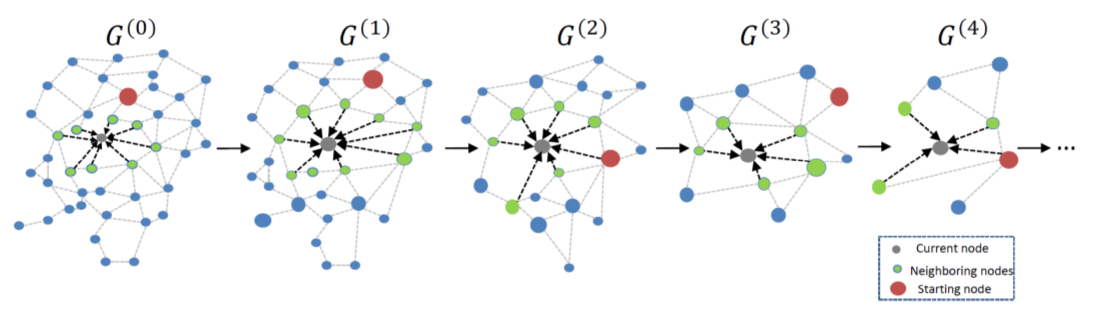Figure 1. An illustration of the structure evolving process of the proposed structure-evolving LSTM model. Starting from an initial graph G (0) , the structure-evolving LSTM learns to evolve the hierarchical graph structures with a stochastic and bottom-up node merging process and then propagates the information on these generated multi-level graph topologies following a stochastic node updating scheme.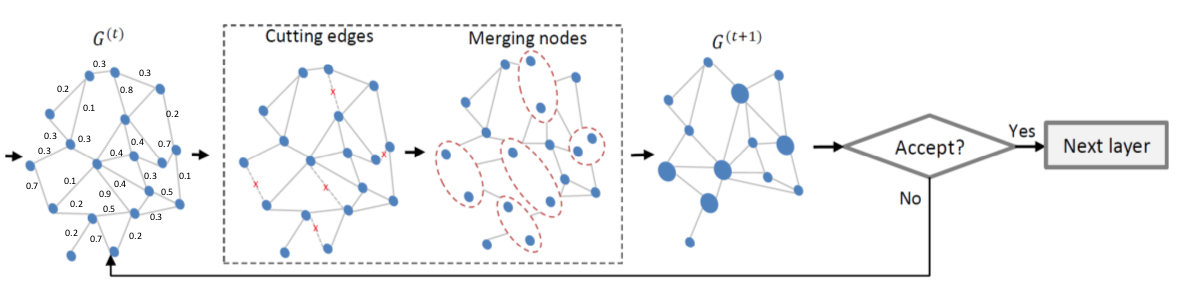Figure 2. Illustration of the stochastic structure-evolving step for evolving a lower-level graph into a higher-level one. Given the computed merging probabilities for all nodes, our structure-evolving step takes several trials to evolve a new graph till the new graph is accepted evaluated by the acceptance probability. A new graph is generated by stochastically merging two nodes with high predicted merging probabilities and thus the new edges are produced. The acceptance probabilities are computed by considering the graph transition cost and the advantage discriminative capability brought by the new graph.Figure 3. Overview of the segmentation network architecture that employs the structure-evolving LSTM layer for semantic object parsing in image domain. Based on the basic convolutional feature maps, five structure-evolving LSTM layers are stacked to propagate information on the stochastically generated multi-level graph structures (i.e., G (0) , G (1) , G (2) , G (3) , G (4) ) where G (0) is constructed as the superpixel
neighborhood graph. The convolutional layers are appended on all LSTM layers to produce the multi-scale predictions, which are then combined to generate the final result.

Experiment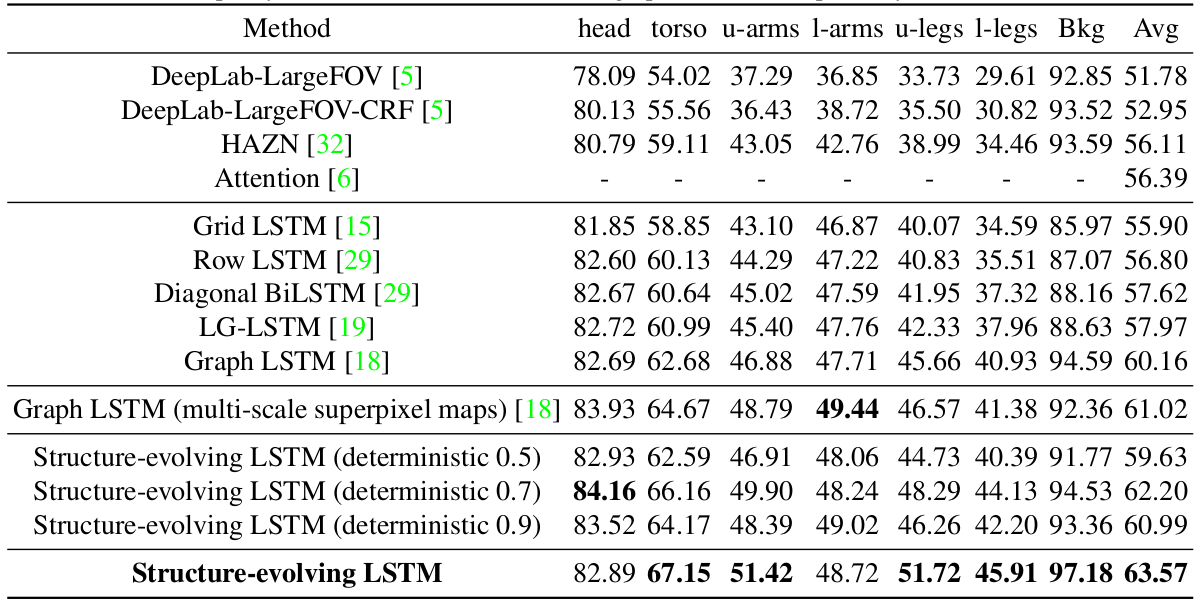Table 1. Comparison of semantic object parsing performance with several state-of-the-art methods on the PASCAL-Person-Part dataset and with other variants of the structure-evolving LSTM model, including using different LSTM structures, the extracted multi-scale super-pixel maps and a deterministic policy with different thresholds for the graph transition, respectively.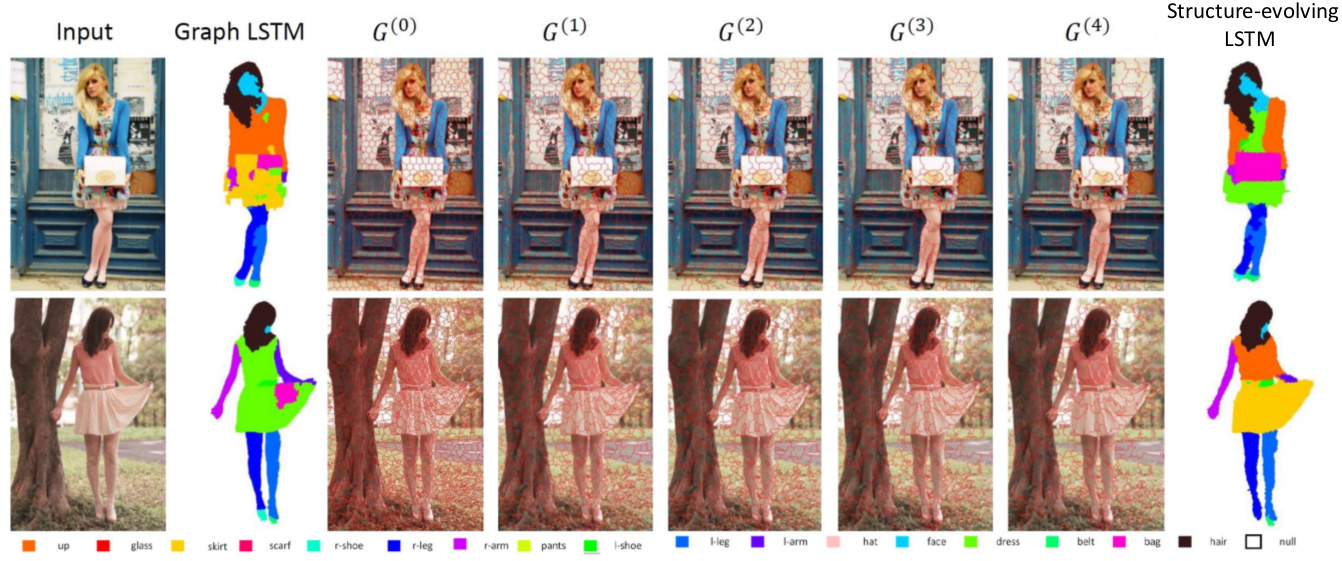Figure 4. Comparison of parsing results of our structure-evolving LSTM and Graph LSTM on ATR dataset and the visualization of the corresponding generated multi-level graph structures. Better viewed in zoomed-in color pdf.

References

 X. Liang, X. Shen, J. Feng, L. Lin, and S. Yan. Semantic object parsing with graph lstm. ECCV, 2016.

 X. Liang, X. Shen, D. Xiang, J. Feng, L. Lin, and S. Yan. Semantic object parsing with local-global long short-term memory. CVPR, 2016.

 X. Chen, R. Mottaghi, X. Liu, S. Fidler, R. Urtasun, et al. Detect what you can: Detecting and representing objects using holistic models and body parts. In CVPR, pages 1979–1986, 2014.

 L.-C. Chen, Y. Yang, J. Wang, W. Xu, and A. L. Yuille. Attention to scale: Scale-aware semantic image segmentation. CVPR, 2016.

 X. Liang, C. Xu, X. Shen, J. Yang, S. Liu, J. Tang, L. Lin, and S. Yan. Human parsing with contextualized convolutional neural network. In ICCV, 2015.